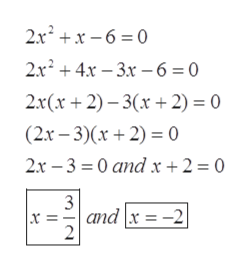Question
2 views

Solve by factoring

check_circle

star
star
star
star
star
1 Rating
Step 1

Given:

Step 2

Solution using factori...help_outlineImage Transcriptionclose2.x +x – 6 = 0 2.x? + 4x – 3x -6=0 2.x(x + 2) – 3(x + 2) = 0 (2x – 3)(x + 2) = 0 2x – 3 = 0 and x + 2 = 0 and x = -2 fullscreen

### Want to see the full answer?

See Solution

#### Want to see this answer and more?

Solutions are written by subject experts who are available 24/7. Questions are typically answered within 1 hour.*

See Solution
*Response times may vary by subject and question.
Tagged in
MathAlgebra

### Equations and In-equations